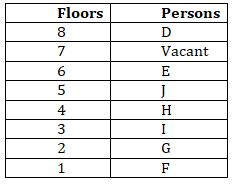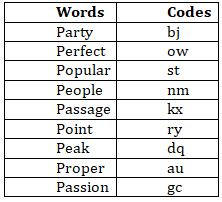Latest Banking jobs   »   Reasoning Ability Quiz For IBPS RRB...

# Reasoning Ability Quiz For IBPS RRB PO, Clerk Prelims 2021- 8th August

Directions (1-5): Study the following information carefully and answer the questions given below:
In a building of a eight floors, seven persons are living on seven different floors such that the first floor is numbered as 1, the floor above is numbered as 2 and so on till the topmost floor which is numbered as 8. One floor remains vacant.
The vacant floor lies above E’s floor. There are two floors between E and I. D lives above the vacant floor. G lives immediately above F. I lives on an odd numbered floor below 4th floor. Number of persons living above H is the same as the number of persons living below H. J lives immediately below E.

Q1. How many persons are living above J’s floor?
(a)Five
(b)Three
(c)Two
(d)One
(e)None

Q2. Which of the following floor is vacant?
(a)Third
(b)Fifth
(c)First
(d)Seventh
(e)Second

Q3. Who among the following lives on the 1st floor?
(a)No one
(b)I
(c)E
(d)F
(e)None of these

Q4. Four of the following five are alike in a certain way and hence form a group, which of the following does not belong to the group?
(a)H
(b)G
(c)E
(d)I
(e)D

Q5.Which of the following is not true?
(a)7th floor is vacant
(b)H lives above G
(c)E lives on an odd numbered floor
(d)D lives immediately above vacant floor
(e)F lives below J

Directions (6-10): Study the following information carefully and answer the questions given below:

5 b n @ 7 w k 2 8 ! m n s % 2 7 d 4 j # v 1 & f z \$ h x £ 2 b c e @ 4 p \$ 9 6 b n

Q6. How many numbers are there which are immediately preceded by a consonant but not immediately followed by a symbol?
(a)One
(b)Two
(c)Three
(d)Four
(e)Five

Q7.Which of the following element is sixth to the right of the seventh element from the left end?
(a)%
(b)s
(c)7
(d)4
(e)None of these

Q8.What should come in place of the question mark (?) in the following series based on the above arrangement?
n@7, k2!, ns7, 4j&, ?
(a)fzh
(b)f\$h
(c)z\$b
(d)fzb
(e)fh2

Q9. How many numbers are there between the thirteenth element from the right end and nineteenth element from the left end?
(a)One
(b)Two
(c)Three
(d)Four
(e)None

Q10. If all the symbols are deleted, then which of the following element is fifth to the left of sixth element from the right end?
(a)x
(b)h
(c)z
(d)f
(e)1

Direction (11-15): Study the following information carefully to answer the given questions:
In a certain code language
“people popular perfect party’’ is coded as “nm st ow bj”
“party passage point peak” is coded as “bj kx ry dq”
“perfect party passage proper” is coded as “ow bj kx au”
“point popular proper passion” is coded as “ry st au gc”

Q11. What is the code for the word “perfect”?
(a)bj
(b)ow
(c)st
(d)nm
(e)au

Q12. What is the code for the word “popular passage”?
(a)nm kx
(b)nm dq
(c)st dq
(d)st kx
(e)ry kx

Q13. If “proper planning” is coded as “au pf” then what could be the possible code for “ planning vacations”?
(a)pf dq
(b)au st
(c)au nm
(d)pf kx
(e)pf it

Q14. What is the code for the word “peak”?
(a)dq
(b)ow
(c)bj
(d)kx
(e)gc

Q15. What could be the possible code for “party preference”?
(a)bj ow
(b)bj kx
(c)au gc
(d)bj lw
(e)st bj

Practice More Questions of Reasoning for Competitive Exams:

###### Target IBPS RRB PO and Clerk Prelims 2021

Solutions

Solutions (1-5):
Sol.S1. Ans. (c)
S2. Ans. (d)
S3. Ans. (d)
S4. Ans. (d)
S5. Ans. (c)

S6. Ans. (b)
Sol. k28, d4j

S7. Ans. (b)
Sol. s

S8. Ans. (c)
Sol. z\$b

S9. Ans. (a)
Sol. 1

S10. Ans. (a)
Sol. x

Solutions (11-15):
Sol.S11. Ans. (b)
S12. Ans. (d)
S13. Ans. (e)
S14. Ans. (a)
S15. Ans. (d)#### Congratulations!Union Budget 2023-24: Free PDF